Courses

# RD Sharma Solutions (Part - 2) - Ex-14.1, Lines and Angles, Class 7, Math Class 7 Notes | EduRev

## Class 7: RD Sharma Solutions (Part - 2) - Ex-14.1, Lines and Angles, Class 7, Math Class 7 Notes | EduRev

The document RD Sharma Solutions (Part - 2) - Ex-14.1, Lines and Angles, Class 7, Math Class 7 Notes | EduRev is a part of the Class 7 Course RD Sharma Solutions for Class 7 Mathematics.
All you need of Class 7 at this link: Class 7

#### Question 15:

One of the angles forming a linear pair is a right angle. What can you say about its other angle?

One angle of a linear pair is the right angle, i.e., 90°.
∴ The other angle = 180°​ - 90° = 90​°

#### Question 16:

One of the angles forming a linear pair is an obtuse angle. What kind of angle is the other?

If one of the angles of a linear pair is obtuse, then the other angle should be acute; only then can their sum be 180°.

#### Question 17:

One of the angles forming a linear pair is an acute angle. What kind of angle is the other?

In a linear pair, if one angle is acute, then the other angle should be obtuse. Only then their sum can be 180°.

#### Question 18:

Can two acute angles form a linear pair?

No, two acute angles cannot form a linear pair because their sum is always less than 180°.

#### Question 19:

If the supplement of an angle is 65°; then find its complement.

Let be the required angle.
Then, we have:
x + 65° = 180°
⇒⇒x = 180° - 65° = 115°

The complement of angle cannot be determined.

#### Question 20:

Find the value of x in each of the following figures.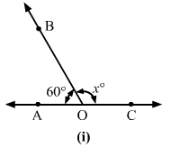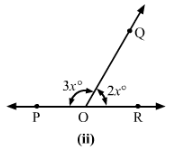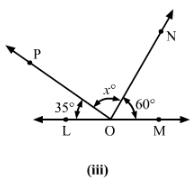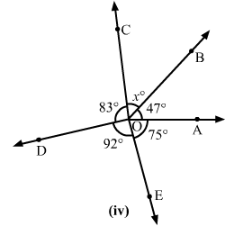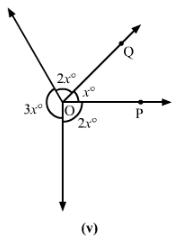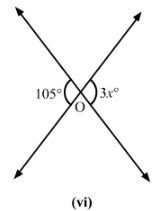(i)
Since ∠BOA+∠BOC=180°                  (Linear pair)
∴ ∠x=180°−∠BOA=180°−60°=120°

(ii)
Since ∠QOP+∠QOR=180°         (Linear pair)

∴2x+3x=180°

⇒5x=180°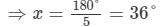(v)
2x°+x°+2x°+3x°=180°⇒8x=180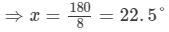(vi)
3x°=105°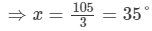#### Question 21:

In Fig. 22, it being given that ∠1 = 65°, find all other angles.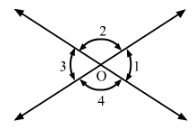∠1=∠3∠1=∠3          (Vertically opposite angles)
∴∠3=65°∴∠3=65°
Since ∠1+∠2=180°       (Linear pair)
∴∠2=180°−65°=115°
∠2=∠4         (Vertically opposite angles)
∴∠4=∠2=115° and ∠3=65°

#### Question 22:

In Fig., OA and OB are opposite rays: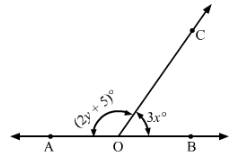(i) If x = 25°, what is the value of y?
(ii) If y = 35°, what is the value of x?

∠AOC + ∠BOC = 180°                   (Linear pair)
⇒(2y+5)+3x=180°
⇒3x+2y=175°

i) If x = 25°, then
3×25°+2y=175°

⇒75°+2y=175°
⇒2y=175°−75°=100°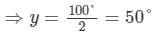(ii) If y = 35°, then
3x+2×35°=175°
⇒3x+70°=175°
⇒3x=175°−70°=105°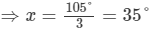#### Question 23:

In Fig., write all pairs of adjacent angles and all the linear pairs.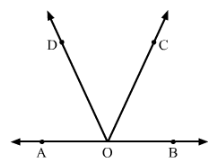∠DOA and ∠DOC
∠DOC and ∠BOC

∠AOD and ∠DOB
∠BOC and ∠AOC

Linear pairs of angles:

∠AOD and ∠DOB
∠BOC and ∠AOC

#### Question 24:

In Fig. 25, find ∠x. Further find ∠BOC, ∠COD and ∠AOD.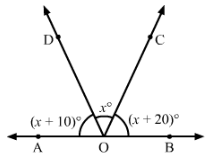∠AOD+∠DOC+∠COB=180°(Linear pair)

(x+10)°+x°+(x+20)°=180°
3x+30°=180°
3x=180°−30°
3x=150°

x=150°/3=50°

∠BOC=x+20°=50°+20°=70°
∠COD=x=50°
∠AOD=x+10°=50°+10°=60°

#### Question 25:

How many pairs of adjacent angles are formed when two lines intersect in a point?

If two lines intersect at a point, then four adjacent pairs are formed, and those pairs are linear as well.

#### Question 26:

How many pairs of adjacent angles, in all, can you name in Fig.?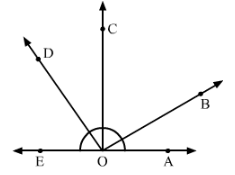There are 10 adjacent pairs in the given figure; they are:
∠EOD and ∠DOC
∠COD and ∠BOC
∠COB and ∠BOA

∠AOB and ∠BOD
∠BOC and ∠COE
∠COD and ∠COA
∠DOE and ∠DOB

∠EOD and ∠DOA
∠EOC and ∠AOC
∠AOB and ∠BOE

#### Question 27:

In Fig., determine the value of x.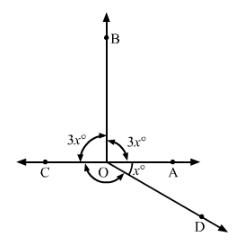∠AOB+∠BOC=180°           (Linear pair)
⇒3x+3x=180°

⇒6x=180°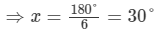#### Question 28:

In Fig., AOC is a line, find x.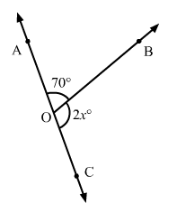∠AOB+∠BOC=180°                (Linear pair)
⇒70°+2x=180°
⇒2x=180°−70°=110°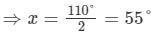#### Question 29:

In Fig., POS is a line, find x.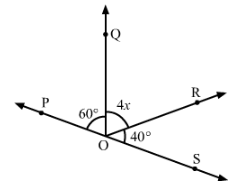∠QOP+∠QOR+∠ROS=180°       (Angles on a straight line)

⇒60°+4x+40°=180°
⇒100°+4x=180°
⇒4x=180°−100°=80°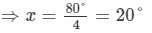#### Question 30:

In Fig., lines l1 and l2 intersect at O, forming angles as shown in the figure. If x = 45°, find the values of yzand u.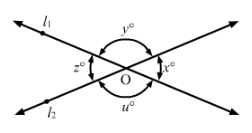∠z=∠x=45°       (Vertically opposite angles)
Now,∠x+∠y=180°      (Linear pair)
⇒∠y=180°−45°=135°
∠u=∠y=135°       (Vertically opposite angles)

#### Question 31:

In Fig., three coplanar lines intersect at a point O, forming angles as shown in the figure. Find the values of xyz and u.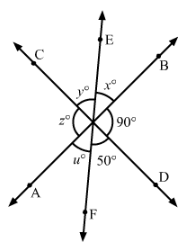∠BOD + ∠DOF + ∠FOA = 180°        (Linear pair)
∴ ∠FOA = ∠= 180°−90°−50°=40
∠FOA=∠x=40°    (Vertically opposite angles)
∠BOD=∠z=90°    (Vertically opposite angles)
∠EOC=∠y=50°    (Vertically opposite angles)

#### Question 32:

In Fig., find the values of xy and z.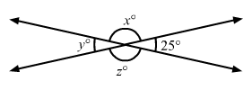∠y=25°       (Vertically opposite angles)
Since ∠x+∠y=180°         (Linear pair)
∴∠x=180°−25°=155°
∠z=∠x=155°        (Vertically opposite angles)

The document RD Sharma Solutions (Part - 2) - Ex-14.1, Lines and Angles, Class 7, Math Class 7 Notes | EduRev is a part of the Class 7 Course RD Sharma Solutions for Class 7 Mathematics.
All you need of Class 7 at this link: Class 7Use Code STAYHOME200 and get INR 200 additional OFF Use Coupon Code
All Tests, Videos & Notes of Class 7: Class 7

### Top Courses for Class 7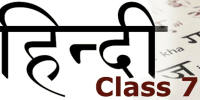## RD Sharma Solutions for Class 7 Mathematics

97 docs

### Top Courses for Class 7Track your progress, build streaks, highlight & save important lessons and more!

,

,

,

,

,

,

,

,

,

,

,

,

,

,

,

,

,

,

,

,

,

,

,

,

,

,

,

,

,

,

;